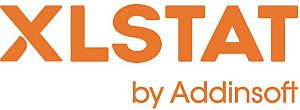# XLSTAT - Univariate Clustering

## Principles of Univariate Clustering

Univariate clustering clusters N one-dimensional observations (described by a single quantitative variable) into k homogeneous classes.

Homogeneity is measured here using the sum of the within-class variances. To maximize the homogeneity of the classes, we therefore try to minimize the sum of the within-class variances.

## Algorithm used in XLSTAT for Univariate Clustering

The algorithm used here is very fast and uses the method put forward by W.D. Fisher (1958). This method can be seen as a process of turning a quantitative variable into a discrete ordinal variable. There are many applications, e.g. in mapping applications for creating color scales or in marketing for creating homogeneous segments.

## Results for Univariate Clustering in XLSTAT

• Class centroids:
This table shows the class centroids for the various descriptors.
• Distance between the class centroids:
This table shows the Euclidean distances between the class centroids for the various descriptors.
• Central objects:
This table shows the coordinates of the nearest object to the centroid for each class.
• Distance between the central objects:
This table shows the Euclidean distances between the class central objects for the various descriptors.
• Results by class:
The descriptive statistics for the classes (number of objects, sum of weights, within-class variance, minimum distance to the centroid, maximum distance to the centroid, mean distance to the centroid) are displayed in the first part of the table. The second part shows the objects.
• Results by object:
This table shows the assignment class for each object in the initial object order.This analysis is available in the XLStat-Basic addin for Microsoft ExcelKovach Computing Services (KCS) was founded in 1993 by Dr. Warren Kovach. The company specializes in the development and marketing of inexpensive and easy-to-use statistical software for scientists, as well as in data analysis consulting.

### Get in Touch

• Email:
sales@kovcomp.com